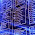## Monday, September 21, 2009

### Generating random passwords with Java

There are times we we need to generate random passwords. For example, we want to send a user a new temporary password instead of an old one, or we just want to generate a strong password for the user, since users usually tend to choose weak password.
We will construct a random password generator written in Java. We will make it a bit smarter than simply producing a sequence of characters and numbers on a given size. The password generator, will be able to produce sequence of different sets of characters from different sizes and combine them together to a random password. For example, suppose we would like to generate 6 characters password combined from the following characters:
• 2 small letters.
• 2 capital letters.
• 2 numbers

An example for such password is: gH1I9s.

our password generator is constructed from 2 classes

• Main class: PasswordGenerator which responsible for generator random passwords according to desired logic.
• Static inner class: PasswordLogic, which defines a set of characters and number of characters to choose from.

The PasswordLogic class is used as an input for the PasswordGenerator class, giving a description of the nature of the password we want to construct. The nature of the password is a set of characters from which we would like to construct the password and the number of characters we would like to use for constructing the password. The PasswordGenerator accepts array of PasswordLogic instances, and use these instances to construct a sequence of characters. We can sum the actions of the PasswordGenerator in these 2 simple steps:

• First we scan each PasswordLogic instance, and produce from its characters array, a random characters set on the desired size.
• Then we order all the characters we produced on a random sequence and combine them to a single string.

Of course, this is a very simple password generator. I find it good enough for most cases. A more complex and sophisticated password generator can be built on this basic one.

Let’s look at the password generator code:

`package com.bashan.blog.password;import java.util.*;public class PasswordGenerator {  private Random random = new Random();  public static final char[] SMALL_LETTERS = {'a', 'b', 'c', 'd', 'e', 'f', 'g', 'h', 'i', 'j', 'k', 'l', 'm', 'n',      'o', 'p', 'q', 'r', 's', 'u', 'v', 'w', 'x', 'y', 'z'};  public static final char[] CAPITAL_LETTERS = {      'A', 'B', 'C', 'D', 'E', 'F', 'G', 'H', 'I', 'J', 'K', 'L', 'M', 'N', 'O', 'P', 'Q', 'R', 'S', 'T', 'U', 'V',      'W', 'X', 'Y', 'Z'};  public static final char[] NUMBERS = {'0', '1', '2', '3', '4', '5', '6', '7', '8', '9'};  private Set<Character> generateChars(char[] charArray, int size) {    Set<Character> chars = new HashSet<Character>();    int arrSize = charArray.length;    for (int i = 0; i < size;) {      char ch = charArray[random.nextInt(arrSize)];      if (!chars.contains(ch)) {        i++;        chars.add(ch);      }    }    return chars;  }  public String generate(PasswordLogic[] passwordLogics) {    // Generate random characters    List<Character> chars = new ArrayList<Character>();    for (PasswordLogic passwordLogic : passwordLogics) {      chars.addAll(generateChars(passwordLogic.chars, passwordLogic.numChars));    }    // Generate random sequence    StringBuffer sb = new StringBuffer();    int size = chars.size();    Set<Integer> sequence = new HashSet<Integer>();    for (int i = 0; i < size;) {      int pos = random.nextInt(size);      if (!sequence.contains(pos)) {        i++;        sb.append(chars.get(pos));        sequence.add(pos);      }    }    return sb.toString();  }  public static class PasswordLogic {    private char[] chars;    private int numChars;    public PasswordLogic(char[] chars, int numChars) {      this.numChars = numChars;      this.chars = chars;    }    public char[] getChars() {      return chars;    }    public void setChars(char[] chars) {      this.chars = chars;    }    public int getNumChars() {      return numChars;    }    public void setNumChars(int numChars) {      this.numChars = numChars;    }  }}`

As you can see, the PasswordGenerator class defines 3 main sets of characters that can be used out-of-the box:

• Upper case characters.
• Small case characters.
• Number characters.

Of course, you can defined your own sets of characters from which you would like to construct passwords.

Here is a small test program showing how the PasswordGenerator can be used in order to construct 2 passwords:

• First password combined from 2 upper case characters, 2 small case characters and 2 numbers:

• Second password combined from 3 numbers, 3 small case letters and 3 characters from the following: ~,!,@,#,\$,%,^,&,*,(,),_,+
`  public static void main(String[] args) {    PasswordGenerator passwordGenerator = new PasswordGenerator();    System.out.println(passwordGenerator.generate(new PasswordLogic[]{        new PasswordLogic(CAPITAL_LETTERS, 2), new PasswordLogic(SMALL_LETTERS, 2),        new PasswordLogic(NUMBERS, 2)}));    System.out.println(passwordGenerator.generate(new PasswordLogic[]{        new PasswordLogic(NUMBERS, 3), new PasswordLogic(SMALL_LETTERS, 3),        new PasswordLogic(new char[] { '~','!','@','#','\$','%','^','&','*','(',')','_','+' }, 3)}));  }`

And sample output for this test program:

`6x5YmKe^+819#an`
Note, that if you don't like looking at so much characters in your eyes, you can simply rewrite this code to work with Strings instead.

1.2.